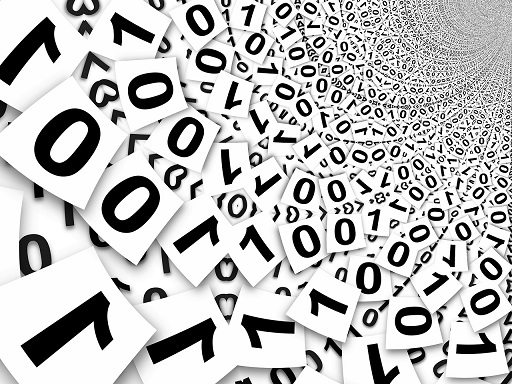# Difference Between Bits and Bytes

## Main Difference – Bits vs. Bytes

Bits and bytes are units of computer memory. The main difference between bits and bytes is that a bit is the smallest unit of computer memory, that has an ability to store a maximum of two different values whereas a byte, composed of 8 bits, can hold 256 different values.

## What is a Bit

Computers are electronic devices, and they only work with discrete values. So, in the end, any type of data that the computer wants to handle is converted to numbers. However, computers do not represent numbers in the same way that we humans do. To represent numbers, we use the decimal system that makes use of 10 digits (0, 1, 2, 3, 4, 5, 6, 7, 8, 9). To represent numbers, modern computers use a binary system made of two digits (0 and 1). “Bit” is the name given to the smallest unit of data that can be represented under this system (Bit stands for “binary digit”). i.e., in terms of binary numbers, a bit consists of either a 0 or a 1. In the electronics that make up the computer, a bit can be represented by having two voltages. An “off” state (0 volts) could represent a binary 0 and an “on” state (having some maximum voltage) could represent a binary 1.

In the binary system, any number can be represented using 0’s and 1’s although binary requires more digits to represent a number than decimal (for instance, the decimal number 123 is represented in binary as 1111011). To express complex data, larger numbers and therefore, more bits are needed. For instance, a colour can be described by how much red, green, and blue go into making up that colour. Under the system that we use, each value for red, green or blue could take up 256 values (0-255). Using binary, then, to represent each red, blue or green value it requires 8 bits (because$2^8=256$). So to represent one colour, it takes up 24 bits in total.In computers, data are represented using 0’s and 1’s.

In the emerging field of quantum computing, the smallest unit of data that can be represented is a qubit. In contrast to our “traditional bits”, qubits make use of quantum mechanical phenomena so that each “bit” is able to represent not just two, but several possible values. Quantum computing is still in its infancy, however once fully developed, quantum computers will be far more powerful than the computers we have today.

## What is a Byte

A byte is a unit of data consisting of 8 bits. Using a byte, one could represent$2^8=256$ different values. For instance, in Java, the data type `byte` is used to represent a number between -128 to 127. In C/C++, the data type `char` is also composed of 8 bits. In C/C++, `char` is used to store a single character. In many computer architectures, a byte is the smallest addressable unit of memory. Traditionally, one single had been represented using 1 byte. Under the original ASCII encoding system, only 7 bits are needed to represent a character, so the last bit would always be 0 (by “last”, I mean the leftmost bit when the number is written down as binary). For instance, the character “B” is represented using 01000010 and the character “b” using 01100010. People who needed to use characters that are not in standard ASCII exploited the unused bit to form their own extended ASCII encoding systems. Nowadays, Unicode encoding is theoretically able to represent more than a million different characters.

Typical files usually have sizes of the order of kilo- or mega- bytes. Here, a kilobyte (KB) consists of 1024 bytes and a megabyte (MB) consists of 1024 kilobytes. Compared to the traditional SI nomenclature, where the subsequently-larger prefixes are defined in terms of multiples of 1000, computers use multiples of 1024 because it is more natural in terms of the binary number system, 1024 being 210 (100000000 in binary).

## Difference Between a Bit and a Byte

### Size of the Unit

A bit is the smallest unit of data that can be represented in computers.

byte consists of 8 bits.

### Values

Using a bit, a maximum of 2 values can be represented.

byte can represent 256 different values.

In many computer architectures, a byte forms the smallest addressable unit of memory. In these systems, the minimum size that a variable can hold is 1 byte.

Image Courtesy:

“binary-system-binary-binary-code-63526” by geralt (Own work) [Public Domain], via Pixabay